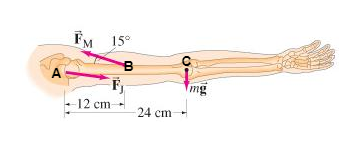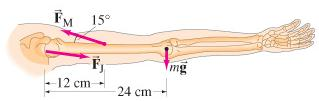# Problem: A. Calculate the force, FM required of the "deltoid" muscle to hold up the outstretched arm shown in the figure. The total mass of the arm is 2.7 kg.B. Calculate the magnitude of the force FJ exerted by the shoulder joint on the upper arm.

###### FREE Expert Solution

Let's label our diagram as follows:Torque:

$\overline{){\mathbit{\tau }}{\mathbf{=}}{\mathbit{r}}{\mathbit{F}}}$

A.

Taking A to be the reference point, the equilibrium of torque about this point can be found by:
rABFmsin(15°) - rACmg = 0###### Problem Details

A. Calculate the force, FM required of the "deltoid" muscle to hold up the outstretched arm shown in the figure. The total mass of the arm is 2.7 kg.

B. Calculate the magnitude of the force FJ exerted by the shoulder joint on the upper arm.